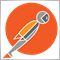# loop through open positions and get max profit47

Hi everyone,

I want to loop through all open positions for one symbol and get the position with max. profit.

This is my code:

```int Positionsforthissymbol=0;

for(int i=PositionsTotal()-1; i>=0; i--)
{
string symbol=PositionGetSymbol(i);

if(Symbol()==symbol)
{
Positionsforthissymbol+=1;
}
}
double profit[];
if(Positionsforthissymbol>0)
{
for(int i=0; i<Positionsforthissymbol; i++)
{
if(PositionSelect(_Symbol)==true)
{
profit[i]=PositionGetDouble(POSITION_PROFIT);
}
}
}
int positionsid=ArrayMaximum(profit,0,WHOLE_ARRAY);```

Does anyone see a mistake?

Thank you in advance!2387

Arrays in MQL are not like lists in high-level languages. You always need to define a size. If you want a dynamically allocated, automatically sized collection then use CArrayDouble instead.47

nicholi shen:
Arrays in MQL are not like lists in high-level languages. You always need to define a size. If you want a dynamically allocated, automatically sized collection then use CArrayDouble instead.

That was the problem, thank you!7

Hello. I can do this. First, are you doing MQL4 or MQL5? I can do it for MQL4/MT4.7

Demosfen:

Hi everyone,

I want to loop through all open positions for one symbol and get the position with max. profit.

This is my code:

Does anyone see a mistake?

Thank you in advance!

As long as you mean MQL4/Metatrader4, let me answer you like this: this is simple...

//Define a function which should return the value you are looking for.

double max_profit(){

double max=-1000000000; /* This is negative 1 billion. Remember -20 is bigger than -35; so your max profit could be -20! */

for(int i=OrdersTotal-1; i>=0; i--){

if(OrderSelect(i, SELECT_BY_POS, MODE_TRADES)==True && OrderSymbol()==Symbol()){

if(OrderProfit()>max){

max=OrderProfit();

}

}

}

return(max);

}7

bagaya:

As long as you mean MQL4/Metatrader4, let me answer you like this: this is simple...

//Define a function which should return the value you are looking for.

double max_profit(){

double max=-1000000000; /* This is negative 1 billion. Remember -20 is bigger than -35; so your max profit could be -20! */

for(int i=OrdersTotal-1; i>=0; i--){

if(OrderSelect(i, SELECT_BY_POS, MODE_TRADES)==True && OrderSymbol()==Symbol()){

if(OrderProfit()>max){

max=OrderProfit();

}

}

}

return(max);

}

So if anyone asks you for maximum profit just give them max_profit() as the answer. The function max_profit() returns the value you are looking for.47

bagaya:
So if anyone asks you for maximum profit just give them max_profit() as the answer. The function max_profit() returns the value you are looking for.

Good solution, thank you

To add comments, please log in or register